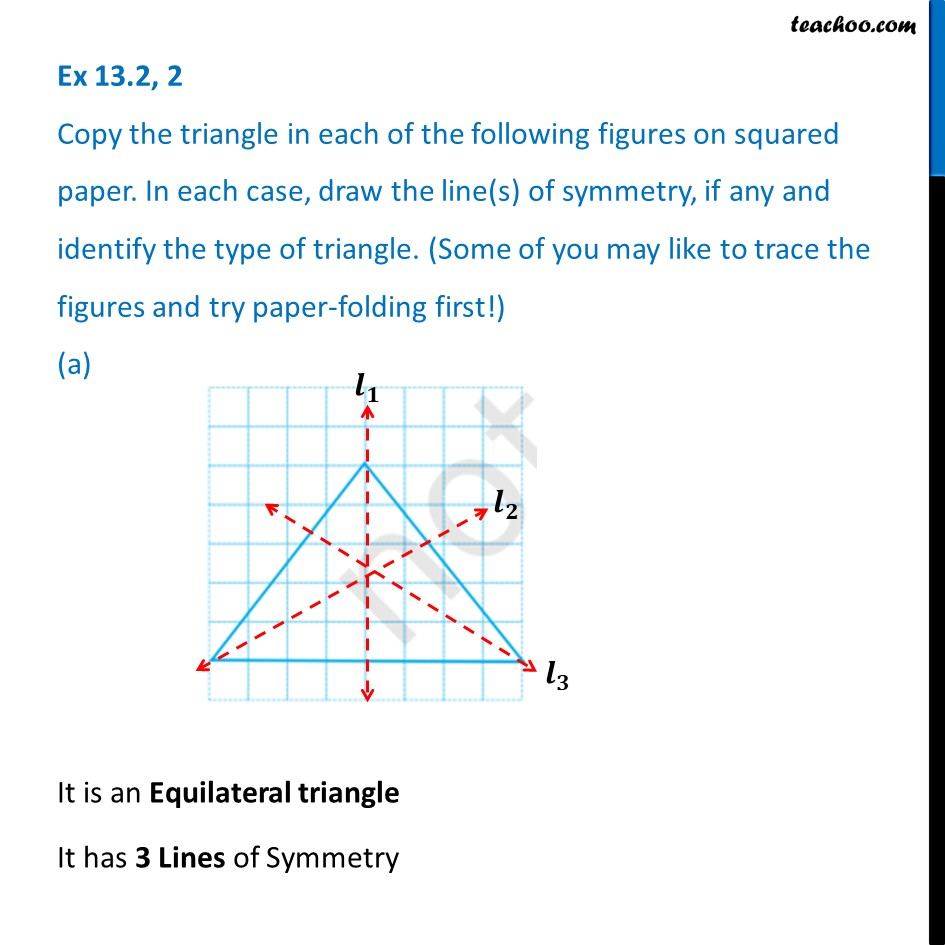1. Chapter 13 Class 6 Symmetry
2. Serial order wise
3. Ex 13.2

Transcript

Ex 13.2, 2 Copy the triangle in each of the following figures on squared paper. In each case, draw the line(s) of symmetry, if any and identify the type of triangle. (Some of you may like to trace the figures and try paper-folding first!) (a) It is an Equilateral triangle It has 3 Lines of Symmetry

Ex 13.2

Chapter 13 Class 6 Symmetry
Serial order wise